Question

# Use the method of variation of parameters to determine the general solution of the given differential equation. y(4)+2y′′+y=3sin(t) Use C1, C2, C3, ... for the constants of integration. Enclose argume...

Use the method of variation of parameters to determine the general solution of the given differential equation.

y(4)+2y′′+y=3sin(t)

Use C1, C2, C3, ... for the constants of integration.

Enclose arguments of functions in parentheses. For example, sin(2x).

 y(t)=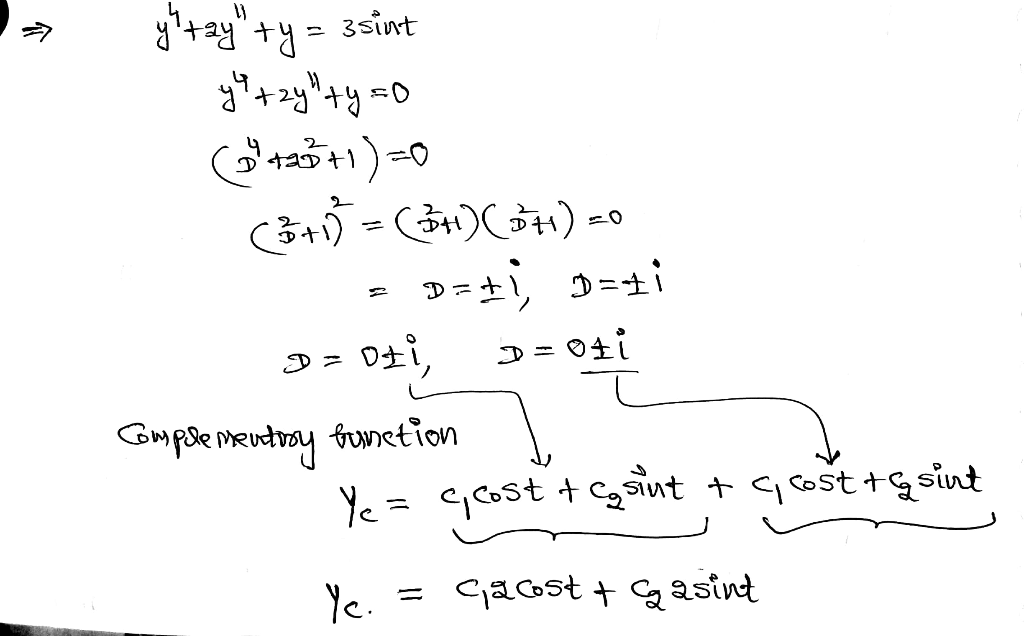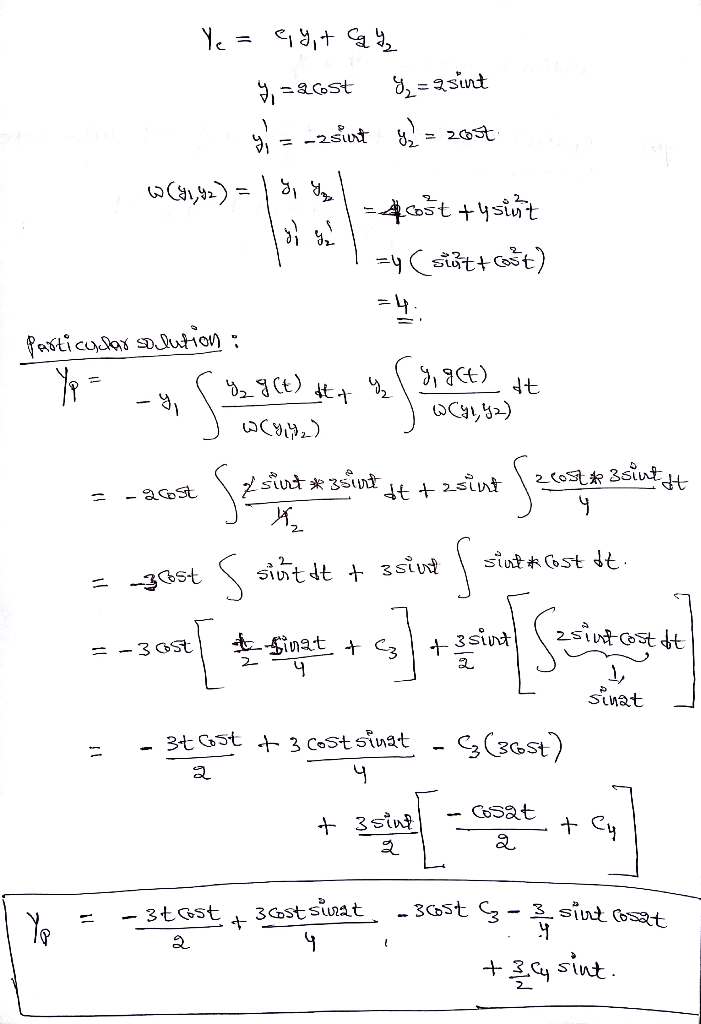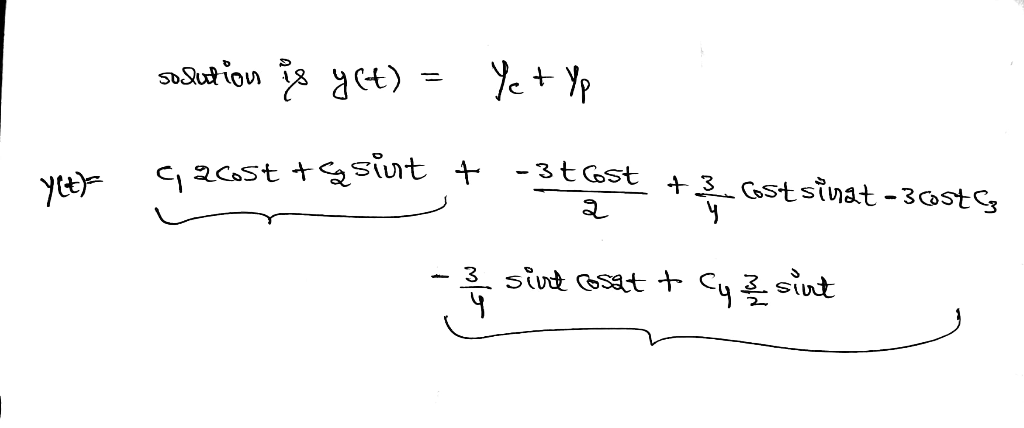#### Earn Coins

Coins can be redeemed for fabulous gifts.

Similar Homework Help Questions
• ### Chapter 4, Section 4.4, Additional Question 01 Use the method of variation of parameters to determine the general solution of the given differential equation. y4 +2y y 11sin (t) Use C1, C2, C3, f...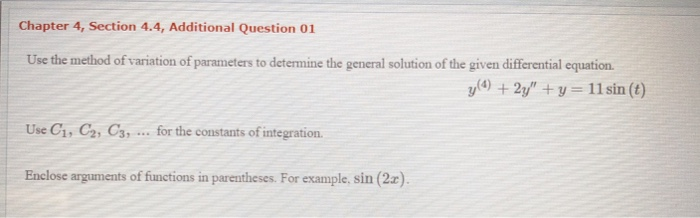Chapter 4, Section 4.4, Additional Question 01 Use the method of variation of parameters to determine the general solution of the given differential equation. y4 +2y y 11sin (t) Use C1, C2, C3, for the constants of integration. Enclose arguments of functions in parentheses. For example, sin (2x) Chapter 4, Section 4.4, Additional Question 01 Use the method of variation of parameters to determine the general solution of the given differential equation. y4 +2y y 11sin (t) Use C1, C2,...

• ### Use the method of variation of parameters to determine the general solution of the given differential...

Use the method of variation of parameters to determine the general solution of the given differential equation. y′′′−2y′′−y′+2y=e6t Use C1, C2, C3, ... for the constants of integration.

• ### Find the general solution of the differential equation y2yy Use C1, C2, for the constants of...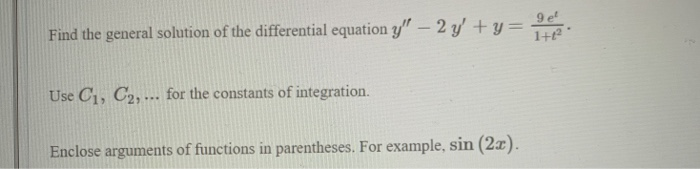Find the general solution of the differential equation y2yy Use C1, C2, for the constants of integration. Enclose arguments of functions in parentheses. For example, sin (2a) 9 el

• ### Use the method of variation of parameters to find the general solution y(t) to the given...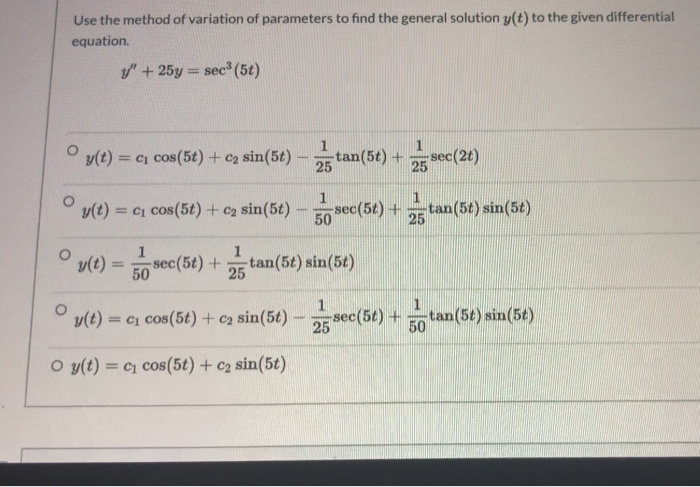Use the method of variation of parameters to find the general solution y(t) to the given differential equation y" + 25y = sec (5t) Oy(t) = ci cos(5t) + c2 sin(5t) tan(5t) + 25 sec(26) 25 y(t) = c cos(5t) + c sin(5t) 1 sec(56) + 50 1 25 tan(5t) sin(5t) VC) = so 1 sec(5t) + 50 1 tan(5t) sin(5t) 25 1 y(t) = ci cos(5t) + c) sin(5t) 2. sec(54) + tan(56) sin(56) 50 O y(t) = C1...

• ### Solve the general solution of the differential equation y'' -2y'+y= -(e^x)/(2x) , using Variation of Parameters...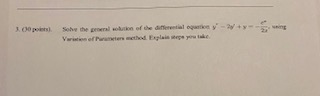Solve the general solution of the differential equation y'' -2y'+y= -(e^x)/(2x) , using Variation of Parameters method. Explain steps please point. She the goal of lo v e

• ### Find the solution of the given initial value problem: y(4) + 2y" + y y(3) (0) y, (0) 0, y', (0) llt + 2; y (0) 1 Enclose arguments of functions in parentheses. For example, sin (2x) Find...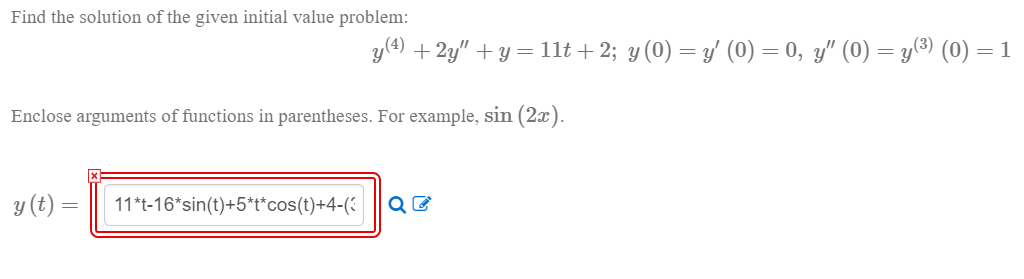Find the solution of the given initial value problem: y(4) + 2y" + y y(3) (0) y, (0) 0, y', (0) llt + 2; y (0) 1 Enclose arguments of functions in parentheses. For example, sin (2x) Find the solution of the given initial value problem: y(4) + 2y" + y y(3) (0) y, (0) 0, y', (0) llt + 2; y (0) 1 Enclose arguments of functions in parentheses. For example, sin (2x)

• ### Find the solution of the given initial value problem in explicit form. y′=(9x)/(y+x^2y), y(0)=−3 Enclose arguments...

Find the solution of the given initial value problem in explicit form. y′=(9x)/(y+x^2y), y(0)=−3 Enclose arguments of functions in parentheses. For example, sin(2x).

• ### 6. Use the method of variation of parameters to find the general solution to the differential...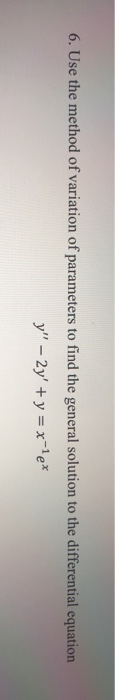6. Use the method of variation of parameters to find the general solution to the differential equation y" - 2y + y = x-le®

• ### Find the general solution of the differential equation. Use C1 and C2 to denote any arbitrary...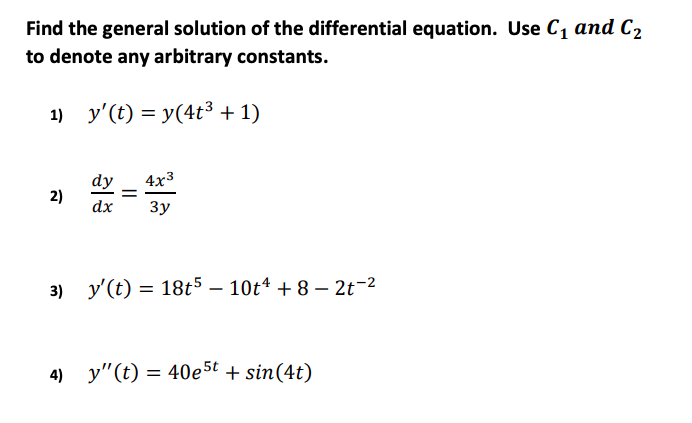Find the general solution of the differential equation. Use C1 and C2 to denote any arbitrary constants. 1) y'(t) = y(4t3 + 1) 3) y'(t) = 18t5 – 10t4 + 8 – 2t-2 4) y"(t) = 40e5t + sin(4t)

• ### Use the method of variation parameters to find the general solution of the differential equation y"...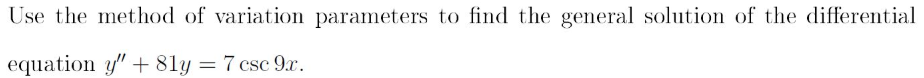Use the method of variation parameters to find the general solution of the differential equation y" + 8y = 7 csc 9x.